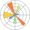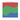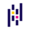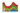# Stacked area Chart

A stacked area chart displays the evolution of a numeric variable for several groups of a dataset. Each group is displayed on top of each other, making it easy to read the evolution of the total, but hard to read each group value accurately. In python, stacked area charts are mainly done thanks to the `stackplot()` function

## ⏱ Quick start

``````# library
import numpy as np
import matplotlib.pyplot as plt

# Create data
x=range(1,6)
y1=[1,4,6,8,9]
y2=[2,2,7,10,12]
y3=[2,8,5,10,6]

# Basic stacked area chart.
plt.stackplot(x,y1, y2, y3, labels=['A','B','C'])
plt.legend(loc='upper left')
``````

##Stacked Area chart with `Matplotlib`

`Matplotlib` is the most common way to build a stacked area chart with Python. The examples below start by explaining to basics of the `stackplot()` function. The also describe the most common type of customization like changing colors, controling group order and more.

##Percent Stacked Area chart with `Matplotlib`

A variation of the stacked area graph is the percent stacked area graph where the value of every groups are normalized at each time stamp. It allows to study the percentage of each group in the whole more efficiently.

Fortunately, the `pandas` library has a `divide()` function that allows to apply this normalization easily.Basic percent stacked area chart

##Stacked Area chart with `Pandas`

`Pandas` is mainly useful to normalize your dataset and build a stacked area chart. Surprisingly, it also provides a `plot.area()`that can be handy to build a stacked area chart.Stacked area chart with Pandas

##Best python stacked area chart examples

The web is full of astonishing charts made by awesome bloggers, (often using R). The Python graph gallery tries to display (or translate from R) some of the best creations and explain how their source code works. If you want to display your work here, please drop me a word or even better, submit a Pull Request!

## Contact

👋 This document is a work by Yan Holtz. You can contribute on github, send me a feedback on twitter or subscribe to the newsletter to know when new examples are published! 🔥